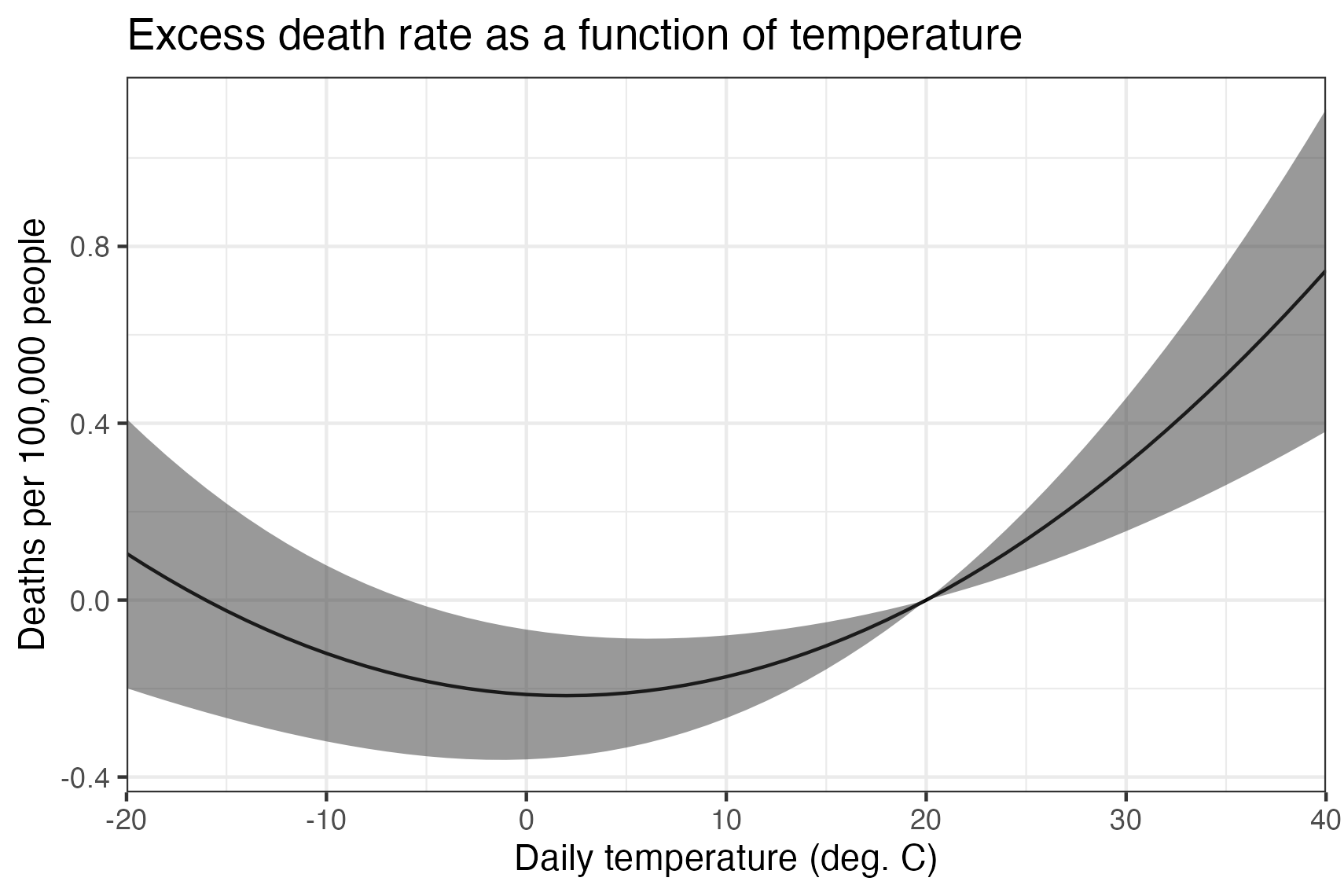# Hands-On Exercise, Step 4: Producing results¶

## Constructing annual polynomials summed over days¶

We have county aggregated data from the previous step, but we also need to sum it to the annual level to match the mortality data. Again, this code should be run from a sister directory to the data directory.

Let’s first turn the data from wide form into long form and label the years.

library(reshape2)

clim2 <- melt(clim[, c(-1:-5)], id.vars='FIPS')
clim2$date <- as.Date("1980-01-01") + as.numeric(gsub("tas_adj|tas_sq", "", clim2$variable))
clim2$year <- as.numeric(substring(clim2$date, 1, 4))


Now we can sum over years. To do this, we will need to base this on the first several characters of the column names (not variable row values).

library(dplyr)

clim3 <- clim2 %>% group_by(FIPS, year) %>% summarize(tas_adj=sum(value[substring(variable, 1, 7) == 'tas_adj']), tas_sq=sum(value[substring(variable, 1, 6) == 'tas_sq']))


## Merging weather and outcome data¶

And now we can merge in the mortality data! This is by county (FIPS code) and year. We also construct the death rate, as deaths per 100,000 people in the population.

df <- read.csv("../data/cmf/merged.csv")

df2 <- df %>% left_join(clim3, by=c('fips'='FIPS', 'year'))

df2$deathrate <- 100000 * df2$deaths / df2$pop df2$deathrate[df2$deathrate == Inf] <- NA  ## Running the regression¶ Let’s run our central regression, relating death rate to temperature. Note that since we do not control for precipitation, temperature in this case includes correlated effects of rainfall. As a result, it is not ideal for future projections. For fixed effects, we use county fixed effects and state trends. More saturated fixed effects should be explored. library(lfe) df2$state <- as.character(floor(df2$fips / 1000)) mod <- felm(deathrate ~ tas_adj + tas_sq | + factor(state) : year + factor(fips) | 0 | fips, data=df2)  ## Plotting the resulting dose-response function¶ To plot the result, we construct evenly sampled temperature from $$-20^\circ$$ C ($$-4^\circ$$ F) to $$40^\circ$$ C ($$104^\circ$$ F). Then we can reconstruct the adjusted temperatures. The normalization we used ensures that all of the reported values are relative to $$20^\circ$$ C, so there are no confidence intervals at this point. For R, to get confidence intervals from felm, we use the predict.felm function from the felm-tools.R library at https://github.com/jrising/research-common/blob/master/R/felm-tools.R plotdf <- data.frame(tas=seq(-20, 40)) plotdf$tas_adj <- plotdf$tas - 20 plotdf$tas_sq <- plotdf\$tas^2 - 20^2

source("felm-tools.R")

preddf <- predict.felm(mod, plotdf, interval='confidence')

plotdf2 <- cbind(plotdf, preddf)

library(ggplot2)

ggplot(plotdf2, aes(tas, fit)) +
geom_line() + geom_ribbon(aes(ymin=lwr, ymax=upr), alpha=.5) +
scale_x_continuous(name="Daily temperature (C)", expand=c(0, 0)) +
ylab("Deaths per 100,000 people") +
ggtitle("Excess death rate as a function of temperature") + theme_bw()